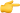Dedicated Serveroffering the top-tier Dedicated Server series for local visitors. Details

### Bash Math Operations ExplainedIn Bash scripting, Bash Math Operations are Explained, and arithmetic operations are crucial. Basic arithmetic operations are necessary for many automation jobs, including converting the CPU temperature to Fahrenheit. Bash is a very straightforward and simple language to learn.

This tutorial shows you several different ways to perform simple math in Bash:

2. Examples of text editors for coding include nano and Vi/Vim.
3. Basic familiarity with Bash scripting.

## Why Do You Need Bash Math Operations Explained Scripting?

Although the primary goal of Bash scripting is not mathematics, being able to perform basic calculations is useful in a variety of situations.

Typical use cases comprise:

1. Number addition, subtraction, multiplication, and division.
2. rounding figures.
3. Numbers that increase and decrease.
4. Unit conversions.
5. Calculations with floating points.
6. Calculating proportions.
7. Utilizing various number bases (binary, octal, or hexadecimal).

Bash Math Operations Explained and arithmetic in Bash scripting assist in doing a quick calculation, producing instant results in the preferred format, depending on the automation requirement.

## Math Commands and Techniques in Bash

Some Linux commands enable the instantaneous execution of simple and complex calculations. Examples using each technique are provided in this section.

### 1: Calculus Expansion

The shell arithmetic expansion method is the preferred one for performing math in Bash. Math expressions are evaluated using the built-in capability, which then returns the outcome. For arithmetic expansions, the syntax is:

• `\$((expression))`

The following is the syntax:

1. `The compound notation that evaluates an expression is (()).`
2. `the result is stored using the variable operator \$.`

As an illustration, add two integers and repeat the outcome:

• `echo \$((2+3))`

When working with Bash scripts, the arithmetic expansion notation is the recommended technique. In Bash, the notation is frequently used in conjunction with if statements and for loops.

### 2: Command awk

Pattern expressions are selected using the awk command. Use the example statement below to execute addition using the awk command:

• `awk 'BEGIN x = 2; y = 3; print 'x + y = '(x + y)'`

The output for the variables x = 2 and y = 3 prints x + y = 5 to the console.

### 3: Command bc

The command-line tool bc command (short for basic calculator) interprets the bc language. To conduct arbitrary precision arithmetic, the application runs as an interactive program or accepts standard input.

To retrieve results, enter an equation from standard input into the command. For instance:

• `bc | echo "2+3"`

The calculation’s outcome is printed in the output.

### 4: Command dc

Reverse Polish notation is supported by the calculator utility known as the dc command (short for desk calculator). The application offers unlimited precision arithmetic and accepts standard input.

To retrieve the result, provide a standard input equation into the program. For instance:

• `dc echo "2 3 + p"`

The print signal is sent to the dc command by the equation’s p.

### 5: Announce Command

Integer computations are supported via the Bash declare command. Add the -I option to declare to utilize it for calculations. For instance:

• `Declare x=2y=3z=x+y`

To observe the outcomes, echo each variable:

• `echo \$x + \$y = \$z`

Each variable is printed to the console in the output.

### 6: Command expr

An old command-line tool for assessing integer arithmetic is the expr command. An illustration of an expr command is as follows:

• `expr 2 + 3`

Run the command to examine the results of the calculation after separating the numbers and the operation sign with spaces.

### 7: Element Command

A command-line tool called factor prints the factors for any positive integer, and the output factors into prime numbers.

Run the following command, for instance, to print the factors of 100.

• `percent 100`

The factored number is displayed in the output.

### 8: Allow Command

The let command in Bash can carry out a number of arithmetic, bitwise, and logical operations. The native command only supports integers.

The let command syntax is demonstrated by the following example:

• `x = 2 + 3 | echo \$x`

Results are printed in the output.

### 9: Command Test

In Linux, the test command analyses conditional expressions and is frequently used in tandem with the if statement in Bash. The test syntax has two different forms:

• `echo \$; test 2 —gt 3?`

As an alternative:

• `echo \$; [2 -gt 3];`

The test command determines if two or (-gt) three is greater. The output is zero (0) if the expression is true and one (1) if it is false.

## Conclusion

You are proficient at using Bash Math Operations Explained scripting to do a variety of calculations.

On Key

## Related PostsDedicated Server Guide### The Center ARK Dedicated Server Not Working

ARK: The Center is a popular survival game that is enjoyed by many players around the world. However, some players have reported issues with theDedicated Server Guide### Golden Eye Source Dedicated Server

Golden Eye Source Dedicated Server (GES DS) is a software application that allows individuals or organizations to host multiplayer game servers for the fan-made mod,Dedicated Server Guide### ARK Dedicated Server Switching to Scorched Earth

An ARK Dedicated Server is a type of server that allows players to host and play the game ARK: Survival Evolved. Scorched Earth is aDedicated Server Guide### How to Get the Center on an ARK Dedicated Server?

ARK: Survival Evolved is a popular survival game that can be played on dedicated servers. These servers allow players to have more control over theirDedicated Server Guide### How to Make a Reign of Kings Dedicated Server?

Reign of Kings is a popular medieval survival game that allows players to build their kingdoms, conquer other kingdoms, and engage in epic battles. The